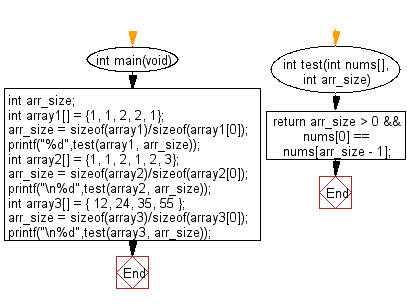﻿ C : Check the first and last elements of an array are equal# C Exercises: Check a given array of integers of length 1 or more and return true if the first element and the last element are equal in the given array

## C-programming basic algorithm: Exercise-34 with Solution

Write a C program to check a given array of integers of length 1 or more and return true if the first element and the last element are equal in the given array.

C Code:

``````#include <stdio.h>
#include <stdlib.h>
int main(void){
int arr_size;
int array1[] = {1, 1, 2, 2, 1};
arr_size = sizeof(array1)/sizeof(array1);
printf("%d",test(array1, arr_size));
int array2[] = {1, 1, 2, 1, 2, 3};
arr_size = sizeof(array2)/sizeof(array2);
printf("\n%d",test(array2, arr_size));
int array3[] =  { 12, 24, 35, 55 };
arr_size = sizeof(array3)/sizeof(array3);
printf("\n%d",test(array3, arr_size));
}
int test(int nums[], int arr_size)
{
return arr_size > 0 && nums == nums[arr_size - 1];
}
``````

Sample Output:

```1
0
0
```

Pictorial Presentation:Flowchart:C Programming Code Editor:

What is the difficulty level of this exercise?

Test your Programming skills with w3resource's quiz.

﻿

## C Programming: Tips of the Day

Why doesn't a+++++b work?

printf("%d",a+++++b); is interpreted as (a++)++ + b according to the Maximal Munch Rule!.

++ (postfix) doesn't evaluate to an lvalue but it requires its operand to be an lvalue.

! 6.4/4 says the next preprocessing token is the longest sequence of characters that could constitute a preprocessing token"

Ref : https://bit.ly/3fdldUT